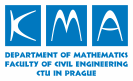﻿ Elective Courses## Elective Courses101MPRS 101MTD 101NMT
 Winter semester 2016/17 Summer semester

# Probability and Statistics

### prof. RNDr. Daniela Jarušková, CSc.

• Course code: 101MPRS
• Hours dotation: 2 + 2
• Credits: 4
• Finals: A (Assessment)

Descriptive statistics and statistical inference. Probability.
Discrete distribution of random variables. Mean, variance, standard deviation.
Continuous distribution, mean, variance, standard deviation, quantiles.
Exponential, normal, log-normal distribution.
Random vectors, dependence of random variables, correlation.
Mean, variance of linear combination of random variables. Two-dimensional normal distribution.
Statistical inference, point and interval estimation of parameters of distribution. Linear regression.

top

# Methods of Time Discretization

### doc. RNDr. František Bubeník, CSc.

• Course code: 101MTD
• Hours dotation: 1 + 1
• Credits: 2
• Finals: A (Assessment)

The course is devoted to a highly versatile and effective method for solving problems containing the time, especially evolutionary problems based on partial differential equations with various boundary and initial conditions. This method represents a modern approach to modeling and solving technical problems, both linear and non-linear, describing processes in various technical areas, such as deflections flat plates, heat conduction, the vibration of beams and plates, reology problems and others. It assumes only a basic knowledge of differential and integral calculus and functional analysis. The course contains basic concepts of functional analysis and variational methods needed to understand the formulation and modeling of technical problems, an overview of solving methods, as well as the theoretical and practical foundations. Problems are physically motivated and examples illustrating and clarifying the theory are given. Weak formulation, generalized and weak solutions are presented. The course is conducted in an accessible form with plenty of examples.

top

# Numerical Methods

### doc. RNDr. Petr Mayer, Dr.

• Course code: 101NMT
• Hours dotation: 1 + 1
• Credits: 2
• Finals: A (Assessment)

The introduction to the basic numerical methods. Great attention is paid to methods for solving systems of linear equations. Further we will study methods of approximation of functions and numerical quadrature. Finally, methods for solving ordinary and partial differential equations, will be studied.

Schedule:
1. Introduction. Representation of numbers and its consequences.
2. Gaussian elimination, LU decomposition.
3. Condition number of matrix. Pivoting strategies.
4. Sparse matrix representation. Reordering.
5. Iterative methods.